Goseeko blog# What are moments?

## Overview

Moments are the statistical tools that we use to describe the characteristics of a distribution. in Statistics, these are the arithmetic means of first, second, third and so on.

Usually in any frequency distribution, four moments we call them first, second, third and fourth moment.

The four moments describe the information about mean, variance, skewness and kurtosis of a frequency distribution.

These are classified as raw and central moments. Raw moments are measured about any arbitrary point A. When we take A as the mean then we get the central moment.

The first raw moment about origin is mean whereas the first central moment is zero. The second raw and central moments are mean square deviation and variance, respectively. The third and fourth moments we use to measure the skewness and kurtosis.

## Moments about mean [Central moments]

If x1, x2, …, xn are the values of the variable under consideration.

The r’th moment μ_r about mean is

Moments about mean for a frequency distribution

If x1, x2, …, xn are the values of a variable x with the corresponding frequencies f1, f2, …, fn respectively then r’th moment  about the mean is defined as

In particular

Hence for any distribution, note it here

Now for r = 1

Hence for any distribution,

For r = 2

Similarly

## Moments about an arbitrary number [Raw moments]

If x1, x2, …, xn are the values of the variable under consideration, the r’th momentabout the number x = a is defined as

In particular

## Moment generating function (mgf)

The moment generating function of the variate x about x = a is defined as the expected value of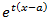and is denoted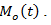Where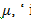‘ is the moment of order  about

Thus the moment generating function about the point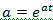moment generating function about the origin.

## Karl Pearson’s coefficients

Skewness: It is the measurement of the symmetry of the given distribution.

In a distribution, if the tail on one side of the mode(top point of a distribution) is fatter than other then we can say that the distribution is skewed.

β_1 – We use this measure for skewness.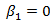for any symmetrical distribution.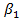does not tell about the direction of skewness whether it is positive or negative.

So that we use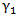Which is the Karl Pearson’s coefficient of skewness.

Kurtosis: It measures the degree of peakedness of a distribution and is the measure of kurtosis.

If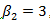, – normal, mesokurtic

If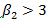, – peaked, leptokurtic

If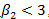, – flat topped, platykurtic

Interested in learning about similar topics? Here are a few hand-picked blogs for you!